## Example Questions

### Example Question #49 : Solid Geometry

If the height of a pyramid was increased by 20% and a side of the square base was decreased 30%, what would happen to the volume of the pyramid?

It would have the same volume

There is a 41% decrease in volume

59% decrease in volume

There is a 59% increase in volume

There is no way to know if it would increase or decrease in volume

There is a 41% decrease in volume

Explanation:

First, you will want to create a pyramid with measurements that are easy to calculate. So, let's say that we have pyramid with a base edge of 10 inches and a height of 10 inches.

So the volume of the original pyramid would be equal to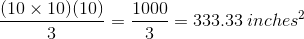The volume of the altered pyramid would be equal to: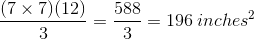To find the relationship between the volume of the altered pyramid relative to the volume of the original pyramid, divide the altered volume by the original volume.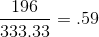The new volume is 59% of the original volume, which means there was a 41% decrease in volume.

### Example Question #50 : Solid Geometry

The Pyramid of Giza has a height of 480 feet. If the length of each side of the base is approximately 756 feet, what is its total surface area? Round to the nearest tenth.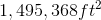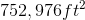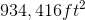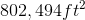Explanation:

If the length of one side is 756 ft, then multiply to find the area of the base.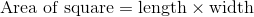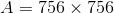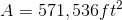Once you've found the area of the base, use the height of the pyramid and half of the side length of the base to determine the length of the side from the apex to the ground using the Pythagorean Theorem.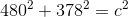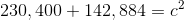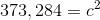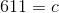Using the side length of the base and the height of each of the triangles that form the pyramid, calculate the area of each triangle, then multiply by 4.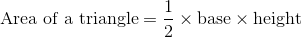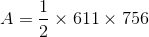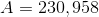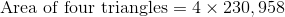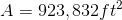Add the surface area of the base to the surface area of the four triangles.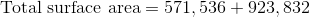### Example Question #1 : Pyramids

The volume of a 6-foot-tall square pyramid is 8 cubic feet. How long are the sides of the base?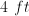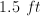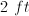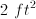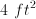Explanation:

Volume of a pyramid is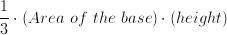Thus: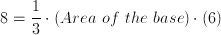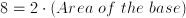Area of the base is.

Therefore, each side is.

### Example Question #1 : How To Find The Volume Of A Pyramid

A right pyramid with a square base has a height that is twice the length of one edge of the base.  If the height of the pyramid is 6 meters, find the volume of the pyramid.

30

6

18

12

24

18

Explanation:

If the height, which is twice the length of the base edges, measures 6 meters, then each base edge must measure 3 meters.

Since the base is a square, the area of the base is 3 x 3 = 9.

Therefore the volume of the right pyramid is V = (1/3) x area of the base x height = 1/3(9)(6) = 18.

### Example Question #51 : Solid Geometry

Find the volume of the pyramid shown below: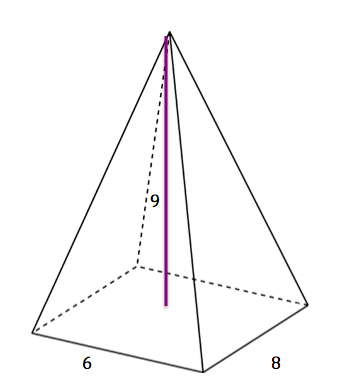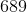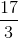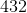Explanation:

The formula for the area of a pyramid is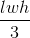. In this case, the length is, the width is, and the height is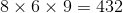and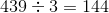### Example Question #2 : How To Find The Volume Of A Pyramid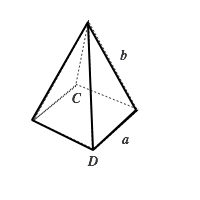Figure not drawn to scale

In the pyramid above, the base is a square. The distance between points C and D is 6 inches and the length of side b is 5 inches. What is the volume of this pyramid?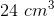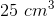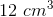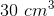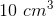Explanation: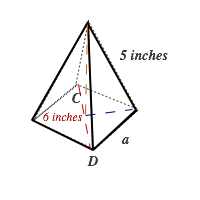To find the volume of a pyramid, you need to use the equation below: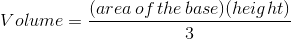To find the height (shown by the yellow line), we can draw a right triangle using the yellow line, blue line and side b (5 inches). Because the hypotenuse is 5 inches, using the common Pythagorean 3-4-5  triple. The blue line is 3 inches and the yellow line (height) is 4 inches. Also, to find side a, we can use the blue line (3 inches) and half of the red line (3 inches)  and the Pythagorean Theorum.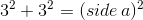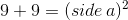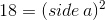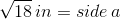Because the base is a square, the area of the base is equal to the square of side a: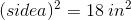Now we plug in these values to find the volume: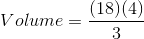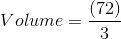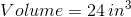### Example Question #3 : Pyramids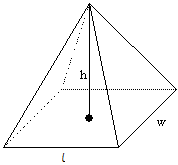Calculate the volume of the rectangular pyramid with height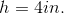, base width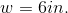, and base length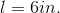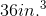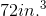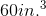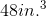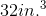Explanation:

The volumeof a rectangular pyramid with height, base width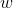, and base lengthis given by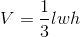.

For this pyramid,, andTo calculate its volume, substitute the values for, andinto the formula: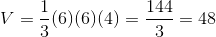Therefore, the volume of the given rectangular pyramid is### All SAT Math Resources